# Cosmological constant

A physical constant characterizing the properties of vacuum, sometimes introduced in the general theory of relativity. Einstein's equations (cf. Einstein equations) including the cosmological constant are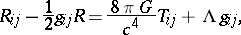whereis the cosmological constant,is the metric tensor,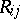is the Ricci tensor,is the curvature of space,is the energy-momentum tensor,is the speed of light, andis the gravitational constant. These equations are the Lagrange equations for the action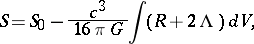where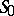is the action for matter anddenotes four-dimensional volume. A. Einstein introduced the cosmological constant in the general theory of relativity  in order to ensure that the equations of the gravitational field admit a spatially homogeneous static solution (the so-called Einstein cosmological model). However, since the advent of Friedmann's evolutionary cosmological model and its experimental verification, the fact that the original Einstein equations have no such solution is not considered a defect of the theory. There are no reliable indications that the cosmological constant is distinct from zero. However, the existence of a sufficiently small cosmological constant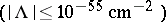does not contradict the observed data or general physical principles.

The existence of a cosmological constant may essentially modify certain steps in the evolution of the most widespread cosmological models (see , Chapt. 4). In this connection, it has been proposed that cosmological models with a cosmological constant should be utilized to explain certain properties of the distribution of quasars (see , , ).

The term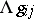in the gravitational field equations may be incorporated in the energy-momentum tensor of vacuum (see ). In this case, vacuum has energy density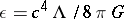and pressure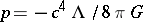, corresponding to the state equation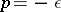. In a theory with a cosmological constant the properties of vacuum already appear in the non-relativistic approximation. Thus, the gravitational potential of a point mass in a theory with a cosmological constant is (see , Chapt. 16)The termis invariant under transformations of the local Lorentz group, corresponding to the principle of Lorentz-invariance of vacuum in quantum field theory. The concept of the cosmological constant as an index of the energy density and pressure of vacuum makes it possible, in principle, to relate the concept of a cosmological constant with the concepts of quantum field theory. There are various formulas that link the value of the cosmological constant to the fundamental physical constants and the age of the Universe (see , Chapt. 24).